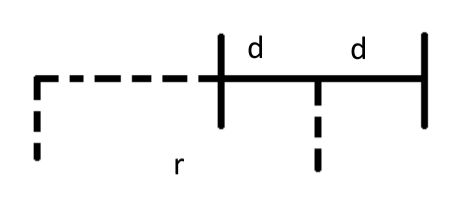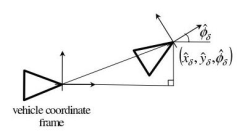0%

## 轮式里程计模型

### 两轮差分底盘的运动学模型

1. 优点

• 结构简单
• 便宜，只需要两个电机（驱动器）
• 模型简单，并且可以实现任何轨迹跟踪
2. 差分模型及运动解算• 考虑两轮在做半径为r的圆周运动，v和w是底盘中心的线速度和角速度，$v{L/R}$ 和 $\omega{L/R}$ 分别对应左/右轮子的线速度和角速度，d为底盘中心离两侧轮子的距离，b为两轮之间的距离（b = 2d）
• 目标：根据两轮的角速度（编码器测出的数据）以及其他参数求出底盘中心（机器人）运动的线速度v和角速度w
• 求解：

• 根据两轮绕圆周中心的角速度相等：

• 因此，

• 根据底盘中心和两轮角速度相等：

• 根据$v = \omega r$

• 整理得

1. 示意图2. 递推公式

• 假设

• 当前位姿（世界坐标系下）为$(x, y, \theta)$
• 运动增量（车体坐标系下）为$(dx, dy, d\theta)$
• 求解更新后的位姿

• 加入噪声后

## 轮式里程计标定

### 线性最小二乘的基本概念

• 求解线性方程组 $Ax = b$，其中

• $A$ 为 $m\times n$ 的矩阵
• $x$ 为 $n\times 1$ 的向量
• 当 $m > n$ 时为超定方程组，方程组无解。实际情况中进行slam时移动机器人约束条件远远多于状态维度，通常为此种情况，此时需要求解最小二乘解
• 最小二乘解

$x^*=(A^TA)^{-1}A^Tb$

### 线性最小二乘在里程计标定中的应用

1. 主要方法包括：

• 直接线性方法：通用性强，实现简单，精度不高（因此实际中比较少直接使用）
• 基于模型的方法：精度高，实现复杂，特异性强（实际中较多使用）
2. 直接线性方法

• 用激光雷达的scan-match数据作为真值$u_i^*$
• 里程计测量数据为$u_i$
• 假设两者成线性关系$u_i^* = Xu_i$，其中：

$X = \begin{bmatrix}x{11} & x{12} & x{13} \x{21} & x{22} & x{23} \x{31} & x{32} & x_{33}\end{bmatrix}$

• 标定过程就是求解$X$的过程（如何矫正里程计测量数据）
• 对于每一组数据，有以下关系：

• 写成矩阵形式有：

• 对于所有n个数据点有：

• 此时可以用最小二乘求解

3. 基于模型方法

• 运动学模型

• 知道 $v$ 和 $\omega$ 之后，可以根据采样时间积分求得机器人状态 $(x(t), y(t), \theta(t))$：

• 根据匀速运动假设（采样时间内机器人保持匀速运动），及运动学模型有：

• 已知两轮的角速度 $\omega_L$ 和 $\omega_R$，需要求解两轮半径（$r_L$ 和 $r_R$）和两轮之间的距离 $b$

• 基本过程：

• 假设激光雷达位于车体的正中心
• 激光雷达的匹配值作为观测值
• 里程计的积分值作为预测值
• 通过最小化预测值和观测值的差即可得到里程计的参数
• 里程计的积分值用$rx$ , $r_y$ , $rθ$ 表示，激光雷达的匹配值用 $sx$ , $s_y$ , $sθ$ 表示
4. 具体求解过程

• 角度积分表达式(单一时间数据点)

• 结合所有时间数据点可以求解 $Ax = b$，可以求得 $J{21}$ 和 $J{22}$

• 在已知 $J{21}$ 和 $J{22}$ 的情况下，对里程计的位置积分可以表示成与参数b成线性关系（$\cos\theta_t$ 的积分可求）：

• 通过罗列所有时间点的约束方程，可以通过最小二乘求解两轮距离 $b$

• 已知 $b, J{21}, J{22}$ 可以求得:

• 至此轮式里程计标定完毕